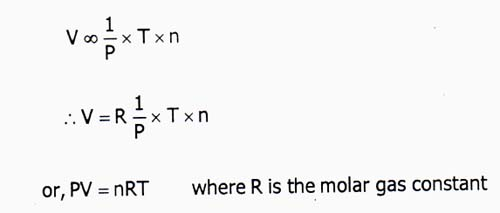# Establish the Ideal Gas Equation

Ideal Gas Equation:

Let pressure, volume and temperature of a fixed mass of gas be P, V and T respectively.

Boyle’s LawAt constant temperature, the volume of a fixed mass of gas is inversely proportional to its applied pressure.

Mathematical form:

V ∞ 1/P  when T is constant ………………(i)

Charle’s Law: At constant pressure, the volume of a given definite mass of a gas increases or decreases by 1/273 part of his volume at 0°C for each degree Celsius rise or fall in temperature.

Alternative form: The volume of a given mass of gas directly proportional to its absolute temperature when pressure remains constant.

Mathematical form:

V ∞ T  when P is constant ……………….(ii)

Avogadro’s Hypothesis: At same temperature and pressure, the equal volume of all gases [elemental or compound] contains the equal number of molecules. It can also be stated as a volume of gases at constant temperature and pressure is directly proportional to their number of moles.

Mathematical form:

V ∞ N when P and T are fixed,

⇒V ∞ n ………………..(iii)

Again, n ∞ N    where n=number of moles and N=number of molecules

From equation (i), (ii) and (iii) we get,

V ∞ (1/P) × T × n

⇒ V = R(1/P) × T × n

or, PV = nRT, Where R is the molar gas constant.

This is the ideal gas equation.